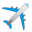Welcome to Microsoft Flight Simulator’s SDK Q&A Platform!

You have questions regarding the SDK? DevMode Tools? SimConnect? You would like to submit an idea for future improvements, seek help or exchange knowledge? You’re in the right place.

Please take a moment to read the platform’s guidelines before you get started!

# question

## Another Atmospheric Model Question

Using the clear weather preset (i.e., not real time weather) to do some flight model work, I have another question regarding the atmospheric modeling. The screenshot below shows the situation shortly after taking off at SKBO (Bogota) with standard sea level pressure, but at a temperature of 42 deg C (using the AGL altitude calculation selection).

The temperature at the airplane's position in the screenshot is 41.4 deg C or 314.55 K. This would mean θ (temperature ratio) = 314.55/288.15 = 1.0916. The ambient pressure at the airplane's position is 734.665 hpa. With sea level pressure being 1013.25 hpa, this means δ (pressure ratio) is 734.665/1013.25 = 0.72504. Since δ=σθ, σ (density ratio)=δ/θ, σ = 0.72504/1.0916 = 0.6642. Since σ is defined as ρ/ρ0, ρ = 0.6642 * 0.00237689 = 0.001579 slugs/ft3. However, MSFS says the ambient density, ρ, is 0.001758 slugs/ft3. Where did this value come from?

A further check on the density calculation can be made using the ideal gas law directly, p = ρRT, or ρ = p/RT. In Imperial units, R = 1716.5619 foot-pounds/slug.oR, p = 734.652 hpa = 1,534.354 lb/ft2, and T = 41.44 C = 566.26 R for the condition above. So ρ = 1534.354/(1716.5619*566.26) = 0.001579 slugs/ft3, the same value calculated above.

Since dynamic pressure is normally calculated from 1/2ρVtrue2, this can have a large impact on the calculated lift and drag forces in a testing environment where preset/customized weather settings are used instead of real weather.

1 comment

Up to 5 attachments (including images) can be used with a maximum of 4.8 MiB each and 23.8 MiB total.

Additional information -- In conducting some additional tests, it appears that this issue is connected with the (high) temperature that I chose for the above mentioned test. This issue does not occur at the default clear skies temperature of 15 C (which is higher than ISA for that elevation), nor when live weather is selected. For those cases, the MSFS ambient density is consistent with the temperature and pressure. Setting an AGL temp of 20 C resulted in a small, but noticeable error in density. Setting the temp of 30 C resulted in a larger density error.
1 Like 1 ·

·

Hello @donstim

We have investigated and identified the issue.
The density value is clamped between 2 bound values and these bounds are too narrow as such high temperatures at high altitude were not anticipated.
The bounds have been modified so that this no longer happens and the fix will come with SU10.

Thank you for the feedback.

Regards,
Sylvain

Up to 5 attachments (including images) can be used with a maximum of 4.8 MiB each and 23.8 MiB total.